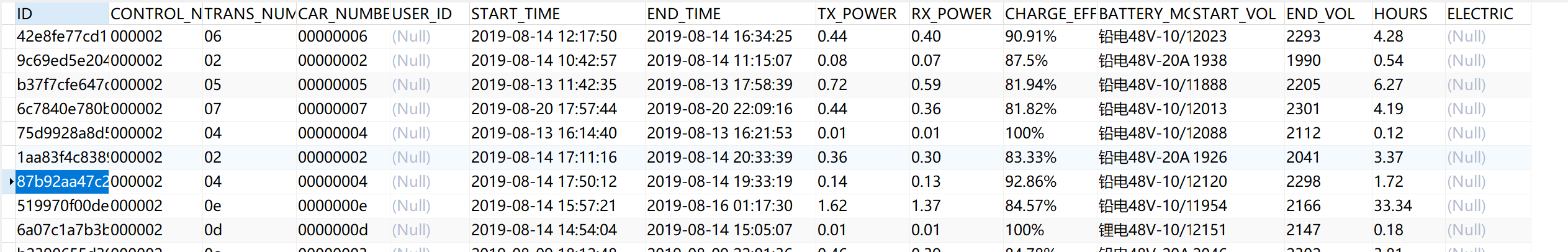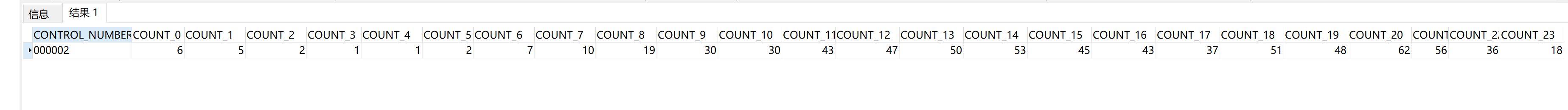• （如果从更严谨的角度看，其实一天也并不等于24小时，或大或小。但这不在本文的讨论范围内）首先阐述这个背景，其实是与今天要说的主题是有关的。我想从另外的角度说说：一天不等于24小时！ 二  大家都知道，...
本文首发于个人微信公众号《andyqian》,期待你的关注！
一
一天等于24小时，已经是不争的事实。（如果从更严谨的角度来看，其实一天也并不等于24小时，或大或小。但这不在本文的讨论范围内）首先阐述这个背景，其实是与今天要说的主题是有关的。我想从另外的角度来说说：一天不等于24小时！
二
大家都知道，研发工程师与产品是有紧密联系的。产品作为需求方，制定需求文档输出给研发，研发则将需求文档以代码的形式转换为用户可使用的软件。这中间需求文档就成了沟通的枢纽，需求文档作为需求的集合，以文字为载体。恰恰文字又是非常有趣的（特别是中文）不同的文字，语境不同，符号不同，表达的意思都相差甚远！我想，这大概就是产品验收时，时而发现研发交付的功能与预期不符的缘故吧！就比如：产品提的需求是：用户登录输入密码错误超过三次则锁定一天？
当你接收到这个需求时，你会怎么想，怎么做呢？
嗯，这里提供两种方案的解读：
方案一：锁定用户1天(自然日)
用户锁定的时间以自然日为单位。例如：用户在23:59分时被锁定，1分钟后则会解锁。已经可以再次尝试登录！
方案二：锁定用户24小时
用户锁定时间以24小时为单位。即：假设用户在23:59分时被锁定，则需要过24小时后解锁。才可以再次尝试登录！
上面两种方案，在实现方式上可能区别不大的，但呈现形式则是完全不一样的。也许产品需要的是第二种，研发人员可能按照第一种理解实现。也许产品需要的是第一种，研发人员可能按照第二种方式实现，甚至都不是。在这里并不是责怪产品或研发的种种不是，但想表达的是：文字的多样性是能造成信息不对称的。
三
当然了，从换算单位上来理解：1天=24小时是毫无疑问的事实。但从某种角度来说，其实它们又是不对等。现在想想来，觉得特别有意思。就如：丹尼尔·卡尼曼在《思考的快与慢》中描述的一样，我们大脑中有两套系统，称之为系统1，系统2。
系统1是：无意识且快速的，不怎么费脑力，完全处于自主控制状态。例如： 1年有12个月，1天有24小时。几乎不需要思考就能给出答案。
系统2则是：需要花费更多脑力来思考。例如：解决一个复杂的数学运算等等。当然，系统1与系统2是相辅相成的。比如：使用系统2解决了多次相同的问题，下次可能就直接通过系统1给出答案了。

相关阅读:
《 张小龙先生与微信 》
《 说说MySQL权限 》
《『不就是』先生 》
《 重构 》扫码关注，一起进步
个人博客: http://www.andyqian.com
展开全文程序
• 表中有开始时间(start_time)和结束时间(end_time) , 我要做的是查询一个日期时间段内(例如2019-10-01 00:00:00至2019-10-31 23:59:59) 的数据 然后再按照一天24小时,统计每条数据的开始和结束的时间段在24小时中的...


ORACLE 将数据按照一天中的24小时进行次数的统计
#首先我的数据表是这样的表中有开始时间(start_time)和结束时间(end_time) , 我要做的是查询一个日期时间段内(例如2019-10-01 00:00:00至2019-10-31 23:59:59) 的数据 然后再按照一天24小时,统计每条数据的开始和结束的时间段在24小时中的分布情况,最后已表中的000002为分组字段,生成一条统计数据 ,然后在前端用echers展示,结果如下:解决方案: (1).按照日期和控制器编号查询出要处理的数据 (2).由于开始和结束时间 有可能两个都在统计的时间段内,也有可能其中一个在要统计的时间段内,所以要再将"(1)"中的数据的开始和结束时间处理下,截掉不在统计时间段内的时间:
CASE WHEN B.START_TIME < TO_DATE( '2019-10-01 00:00:00', 'yyyy-mm-dd hh24:mi:ss' ) THEN TO_DATE( '2019-10-01 00:00:00', 'yyyy-mm-dd hh24:mi:ss' ) ELSE B.START_TIME END

CASE WHEN B.END_TIME > TO_DATE( '2019-11-01 23:59:59', 'yyyy-mm-dd hh24:mi:ss' ) THEN TO_DATE( '2019-11-01 23:59:59', 'yyyy-mm-dd hh24:mi:ss' ) ELSE B.END_TIME END

(3) 因为我是要按照24小时来统计,所以我这里将时间又处理了下,只取24小时制的小时数,使用:
TO_CHAR(START_TIME,'HH24')

(4) 然后我开始从0-23点挨个统计,使用的方法如下,这是统计数据在0点-1点占用的次数,以此类推
 COUNT( CASE WHEN ( A.START_TIME <= 00 AND A.END_TIME >= 00 ) THEN 1 END ) AS count_0,

下面是全部的完整sql 做个记录,你们看看还有没有更好的方法呀
SELECT
CONTROL_NUMBER,
COUNT( CASE WHEN ( A.START_TIME <= 00 AND A.END_TIME >= 00 ) THEN 1 END ) AS count_0,
COUNT( CASE WHEN ( A.START_TIME <= 01 AND A.END_TIME >= 01 ) THEN 1 END ) AS count_1,
COUNT( CASE WHEN ( A.START_TIME <= 02 AND A.END_TIME >= 02 ) THEN 1 END ) AS count_2,
COUNT( CASE WHEN ( A.START_TIME <= 03 AND A.END_TIME >= 03 ) THEN 1 END ) AS count_3,
COUNT( CASE WHEN ( A.START_TIME <= 04 AND A.END_TIME >= 04 ) THEN 1 END ) AS count_4,
COUNT( CASE WHEN ( A.START_TIME <= 05 AND A.END_TIME >= 05 ) THEN 1 END ) AS count_5,
COUNT( CASE WHEN ( A.START_TIME <= 06 AND A.END_TIME >= 06 ) THEN 1 END ) AS count_6,
COUNT( CASE WHEN ( A.START_TIME <= 07 AND A.END_TIME >= 07 ) THEN 1 END ) AS count_7,
COUNT( CASE WHEN ( A.START_TIME <= 08 AND A.END_TIME >= 08 ) THEN 1 END ) AS count_8,
COUNT( CASE WHEN ( A.START_TIME <= 09 AND A.END_TIME >= 09 ) THEN 1 END ) AS count_9,
COUNT( CASE WHEN ( A.START_TIME <= 10 AND A.END_TIME >= 10 ) THEN 1 END ) AS count_10,
COUNT( CASE WHEN ( A.START_TIME <= 11 AND A.END_TIME >= 11 ) THEN 1 END ) AS count_11,
COUNT( CASE WHEN ( A.START_TIME <= 12 AND A.END_TIME >= 12 ) THEN 1 END ) AS count_12,
COUNT( CASE WHEN ( A.START_TIME <= 13 AND A.END_TIME >= 13 ) THEN 1 END ) AS count_13,
COUNT( CASE WHEN ( A.START_TIME <= 14 AND A.END_TIME >= 14 ) THEN 1 END ) AS count_14,
COUNT( CASE WHEN ( A.START_TIME <= 15 AND A.END_TIME >= 15 ) THEN 1 END ) AS count_15,
COUNT( CASE WHEN ( A.START_TIME <= 16 AND A.END_TIME >= 16 ) THEN 1 END ) AS count_16,
COUNT( CASE WHEN ( A.START_TIME <= 17 AND A.END_TIME >= 17 ) THEN 1 END ) AS count_17,
COUNT( CASE WHEN ( A.START_TIME <= 18 AND A.END_TIME >= 18 ) THEN 1 END ) AS count_18,
COUNT( CASE WHEN ( A.START_TIME <= 19 AND A.END_TIME >= 19 ) THEN 1 END ) AS count_19,
COUNT( CASE WHEN ( A.START_TIME <= 20 AND A.END_TIME >= 20 ) THEN 1 END ) AS count_20,
COUNT( CASE WHEN ( A.START_TIME <= 21 AND A.END_TIME >= 21 ) THEN 1 END ) AS count_21,
COUNT( CASE WHEN ( A.START_TIME <= 22 AND A.END_TIME >= 22 ) THEN 1 END ) AS count_22,
COUNT( CASE WHEN ( A.START_TIME <= 23 AND A.END_TIME >= 23 ) THEN 1 END ) AS count_23
FROM
(
SELECT
B.CONTROL_NUMBER,
TO_CHAR( CASE WHEN B.START_TIME < TO_DATE( '2019-10-01 00:00:00', 'yyyy-mm-dd hh24:mi:ss' ) THEN TO_DATE( '2019-10-01 00:00:00', 'yyyy-mm-dd hh24:mi:ss' ) ELSE B.START_TIME END, 'HH24' ) AS START_TIME,
TO_CHAR( CASE WHEN B.END_TIME > TO_DATE( '2019-10-31 23:59:59', 'yyyy-mm-dd hh24:mi:ss' ) THEN TO_DATE( '2019-10-31 23:59:59', 'yyyy-mm-dd hh24:mi:ss' ) ELSE B.END_TIME END, 'HH24' ) AS END_TIME
FROM
H_CHARGE_STATISTIC B
WHERE
B.CONTROL_NUMBER = '000002'
AND B.START_TIME IS NOT NULL
AND B.END_TIME IS NOT NULL
AND B.TX_POWER != 0.00
AND B.RX_POWER != 0.00
AND (( B.START_TIME BETWEEN TO_DATE( '2019-10-01 00:00:00', 'yyyy-mm-dd hh24:mi:ss' ) AND TO_DATE( 2019-10-31 23:59:59', 'yyyy-mm-dd hh24:mi:ss' ) )
OR ( B.END_TIME BETWEEN TO_DATE( '2019-10-01 00:00:00', 'yyyy-mm-dd hh24:mi:ss' ) AND TO_DATE( '2019-10-31 23:59:59', 'yyyy-mm-dd hh24:mi:ss' ) ) )
) A
GROUP BY
CONTROL_NUMBER

展开全文• 因为业务需求我也是查了百度，有...mysql中查询一天中每一个小时的记录数量 select count(*) as orderCounts from ec_order o WHERE o.order_type=1 AND o.order_time &amp;gt;= str_to_date('2018-04-11 00:0...
因为业务需求我也是查了百度，有给出一些sql，我现在再总结一下  我这里是比较两个时间，所以会使用到两个sql  mysql中查询一天中每一个小时的记录数量
select count(*) as orderCounts from ec_order o WHERE o.order_type=1
AND o.order_time  >= str_to_date('2018-04-11 00:00:00','%Y-%m-%d %T')
AND o.order_time < str_to_date('2018-04-11 23:59:59','%Y-%m-%d %T')
group by HOUR(o.order_time)

select count(*) as payCounts from ec_order o WHERE
o.order_type=1 and o.order_status=1 and o.pay_status=1
AND o.pay_time  >= str_to_date('2018-04-11 00:00:00','%Y-%m-%d %T')
AND o.pay_time < str_to_date('2018-04-11 23:59:59','%Y-%m-%d %T')
group by HOUR(o.order_time)
应该很郁闷，这里为什么使用一个时间进行分组，是因为这里是进行比较，比较就需要有一个共同的点才能进行比较，不然就没有意义，所以这里都根据一个时间进行分组
mysql查询一天中每半个小时记录的数量
SELECT HOUR(e.time)as Hour,FLOOR(MINUTE(e.time)/30) as M, COUNT(*) as Count
FROM error_log e
WHERE e.date = '2017-09-02'
GROUP BY FLOOR(MINUTE(e.time)/30),HOUR(e.time)
ORDER BY Hour(e.time);
展开全文•  to_date('2017-09-01', 'yyyy-mm-dd hh:mi:ss')+ rownum/24 dtime  from dual  connect by rownum  ) a  where a.rn =(select round(dbms_random.value(1,24)) from dual);  end loop;    ...
--1.创建测试表 create table test (dtime date);  --2.循环插入测试数据 begin      for i in 1..50000 loop      insert into test      select *    from (select rownum rn,                to_date('2017-09-01', 'yyyy-mm-dd hh:mi:ss')+ rownum/24 dtime                from dual             connect by rownum<25             ) a       where a.rn =(select round(dbms_random.value(1,24)) from dual);    end loop;        commit;   end;  --3.查询结果 select to_char(dtime,'hh24') hour,count(*) as cnt    from test  group by to_char(dtime,'hh24')
order by to_char(dtime,'hh24');

展开全文测试
• 参考：mysql group by分组查询统计数据时，在没有数据时如何自动补0 最终我自己实现的sql如下： SELECT a.hour hour, ifnull(b.count, 0) count FROM ( SELECT 0 hour UNION ALL SELECT 1 hour UNION ALL SELECT 2 ...sql mysql
• //获取某时刻过去的24小时 public static Date getOneDayBefore (Date dateEnd){ Calendar date = Calendar.getInstance(); date.setTime(dateEnd); date.set(Calendar.DATE, date.get(Calendar.DATE) - 1...
• 24小时的时间每两个小时分成个时间段，分成了12条数据oracle sql
• 获取一天24小时每小时的订单数量，没有的置为0   date_tool为统计工具表date值为1~31， hours值为 0~24 month 值为1~12 字段类型可根据自己需求改变 CREATE TABLE date_tool ( TOOL_ID int(10) ...数据库 sql语句
• 标题：一名「软件测试工程师的一天」 副标题：软件测试工程师的一天，工作内容是什么？测试工程师，每天在忙什么 ？ 作者：IDO老徐 8:00 被连着3个闹钟，从睡梦中催醒 。 9:00 在床上磨蹭了一个小时，被最后一个闹钟...软件测试 脚本语言 java makefile 编程语言mysql 时间转换
• 一天24小时钟表中时针，分针和秒针重合了多少次1:05之后有一次 2:10之后有一次 3:15之后有一次 4:20之后有一次 5:25之后有一次 6:30之后有一次 7:35之后有一次 8:40之后有一次 9:45之后有一次 10:50之后有一次 12:00...
• 思路：利用时针与分针的夹角计算。参考：上篇文章 因为电子钟表，表针的跳动不是连续的，譬如分钟每次跳动6度，时针每次跳动0.5度。而编写程序时变量h小时，m分钟每次增量为1，所以我们设夹角gap为（-6~6之间）...
• 根据需求 比如我这里是需要展示一天24小时，且只能展示半点和整点。可以把时间轴等分成48份，这里max取值上限可以设为48，步长为1 重点是自定义的格式化处理函数 formatTooltip (val) { let hour = 0 let min = 0...javascript
• 我用ECharts主要是按显示采集的时间序列数据，并且需要固定展示24小时的数据。 通过ECharts3的时间轴，我们可以把个采集的设备数据呈现在图上面，可是如何漂亮呈现固定的24小时的数据，笔者是通过对ECharts...ECharts3
• SELECT * from 表名 where timestampdiff(MINUTE,时间字段,NOW()) < 24*60; 或者 SELECT * from 表名 where 时间字段>=(NOW() - interval 24 hour)sql
• 本文转载自腾讯网，昨晚（2月10日），“地球自转加快一天已不足24小时”登上微博热搜，达到了“沸”的热度。到底是怎么回事？ 据央视新闻，研究显示，从2020年年中以来，地球的自转速率呈加快趋势，自转速度已达...
• var todayYear=(new Date()).getFullYear(); var todayMonth=(new Date()).getMonth(); var todayDay=(new Date()).getDate(); var todayTime=(new Date(todayYear...angularjs javascript
• i < 24; i++) { Calendar cal = Calendar.getInstance(); cal.setTime(date); cal.add(Calendar.HOUR, 1); date = cal.getTime(); String s1 = format.format(date); dates.add(s1); }   为了统计...
• 一天24小时之中，时钟的时针、分针和秒针完全重合在一起的时候有几次？都分别是什么时间？你怎样算出来的？ 答案：只有两次 思路： 假设时针的角速度是ω（ω=π/6每小时），则分针的角速度为12ω，秒针的...
• 需求：由于查询时候可能要按小时来查询，如果某个小时没有数据就显示为0 。 解决方案： ##当天时间段 ##当天时间段 SELECT DATE_FORMAT( DATE_SUB( DATE_FORMAT( NOW(),'%Y-%m-%d'),INTERVAL ( -(@i:=@i+1) ) HOUR...mysql
• 22,23 union all select 23,24 ) aa  where datediff(day, '2016-12-09', DtTime) = 0 and InOut = '1' group by right(100 + a, 2) + ' -- ' + right(100 + b, 2) + ' ' order by DtTime asc
• 其中有一个需求是获取某个给定的时间段内，每一天的日志数据，比如说要获取从2018-02-02 09:18:36到2018-03-05 23:18:36这个时间段内，统计出每一天的日志数据，一般情况下，看到这种需求都是考虑使用函数搞定，...mysql
• function cookieGO(name) { var today = new Date(); var expires = new Date(); expires.setTime(today.getTime() + 1000*60*60*24); setCookie("cookievalue", name, expires); }cookie 本地存储 js弹框
• $now = time();$start = strtotime('-1 days'); for ($i=$start; $i&lt;=$now; $i+=3600) //3600秒是按每小时生成条，如果按或者月份换算成秒即可 { ... • date_default_timezone_set("... //获得往前推24小时的时间点。 for ($i=$start;$i&lt;=$now;$i+=3600) //3600秒是按每小时生成条，如果按或者月份换算成秒即可 { $date[] = dat...php strtotime • x轴00:00到23:00,24个点,每个小时之间有60条数据,1小时到2小时之间有60个点那样的....echarts3.0 • ## mysql统计24小时数据 千次阅读 2019-09-17 15:55:43 实例2:统计24小时,每个小时没有的补0 SELECT t1.HOUR date, COUNT( t2.HOUR ) dateCount FROM ( SELECT DATE_FORMAT( @cdate := DATE_ADD( @cdate, INTERVAL - 1 HOUR ), '%y-%m-%d %H' ) HOUR FROM ( ... • 项目中需要按当前时间返回（比如...24小时 &lt;?php date_default_timezone_set('PRC');//设置时区$data = date('H:i',time()); var_dump(\$data); 12小时 &lt;?php date_default_timezone_set('PRC');//...
• 文章目录踩点获取所有城市的链接爬取单个城市24小时气温数据处理数据可视化 踩点 首先进入网站 http://www.cma.gov.cn/ 一直往下滑，找到气象服务这里，随便点击个城市 会发现它打开了个新的标签页，进去...python 可视化 爬虫
• 格式里的时如果用hh表示用12小时制，HH表示用24小时制。MM必须是大写! -- 这样有点晕：   字母 日期或时间元素 表示 示例 G Era 标志符 Text AD y ...java date...